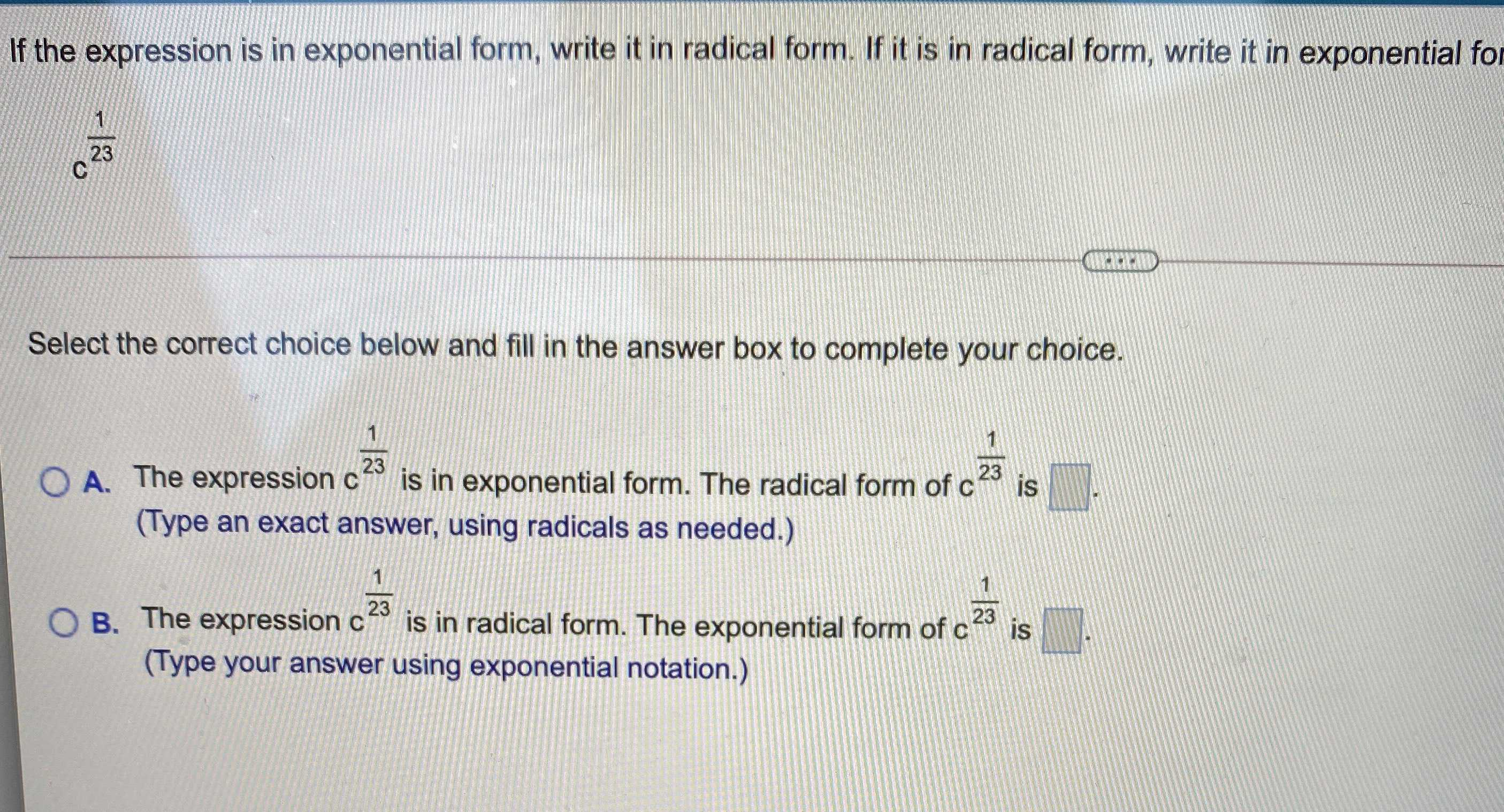### Still have math questions?

Algebra
QuestionIf the expression is in exponential form, write it in radical form. If it is in radical form, write it in exponential fol

$$c ^ { \frac { 1 } { 23 } }$$

Select the correct choice below and fill in the answer box to complete your choice.

A. The expression c $$c ^ { \frac { 1 } { 23 } }$$ is in exponential form. The radical form of $$c ^ { \frac { 1 } { 23 } }$$ is

B. The expression c $$c ^ { \frac { 1 } { 23 } }$$ is in radical form. The exponential form of $$c ^ { \frac { 1 } { 23 } }$$ is
blank = $$\sqrt{c}$$